## You need to test, we're here to help.

You need to test, we're here to help.

## 06 February 2023

### New PCIe 6.0 Compliance Pattern Measurements

PCI Express® 6.0 features significant changes from PCIe® 5.0. In particular, PCIe 6.0 achieves its 64-GT/s data rate, double that of PCIe 5.0, by moving from non-return-to-zero (NRZ) signaling to four-level pulse-amplitude-modulation (PAM4) signaling. Consequently, PCIe 6.0 requires some new test methodologies and patterns, including a new PAM4 Compliance Pattern that finds use in multiple measurements.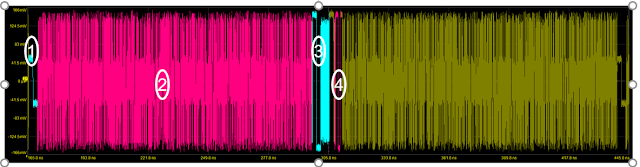Figure 1. The new PCIe 6.0 Compliance Pattern signal. Click any image to enlarge.

The new Compliance Pattern is used for calculating signal to noise and distortion ratio (SNDR), as well as ps21TX (the package insertion loss) and the transmitter ratio of level mismatch (RLM). In addition, it is used to measure transmitter equalization coefficients.

### What's in the Compliance Pattern Signal

The new Compliance Pattern signal (shown in Figure 1 above) contains low-frequency patterns, such as strings of 64 0s, 1s, 2s and 3s, enabling a long run of each of the voltage levels found in PAM4. It also contains a big section of pseudorandom binary sequence (PRBS) data using PAM4.

• Section 1 contains a long run of 2s and 1s.
• Section 2 is the big section containing PRBS data.
• Section 3 contains a long run of 3s and 0s followed by a clock pattern.
• Section 4 contains another long run of 3s and 0s.

More PRBS data follows section 4, and the Compliance Pattern signal repeats itself. One or more of these sections in the Compliance Pattern signal can assist in the calculation of SNDR and other parameters.

### SNDR Measurement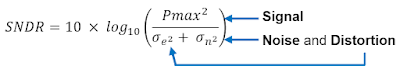Figure 2. SNDR equation variables.

SNDR refers to the Signal to Noise and Distortion Ratio. Figure 2 shows the breakdown of the SDNR equation in terms of the variables Pmax (the signal strength), 𝜎n (noise) and 𝜎e (distortion).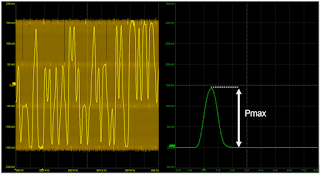Figure 3. Pmax measurement.
The Pmax component of SNDR is calculated by using a pulse response, which is an extracted linear model of the transmitter signal. The pulse response is measured at the transmitter to describe the behavior of the transmitter as a filter if you apply the filter to a 1-UI pulse. Pmax is the maximum amplitude of the extracted pulse response (Figure 3), and it can be affected by various factors such as the channel bandwidth.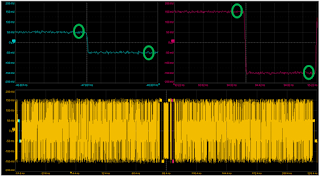Figure 4. Noise variable 𝜎n measurement.

The noise component of the signal, notated 𝜎n, is measured on the 61st UI of the 64-UI-long runs of 0s, 1s, 2s and 3s in the Compliance Pattern signal (Figure 4). The 61st UI is chosen for this measurement because, in theory, the signal has had plenty of time to settle down to its equilibrium state by the time the 61st UI arrives.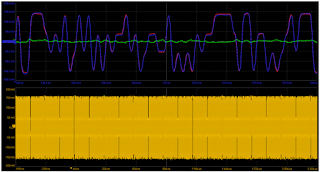Figure 5. Distortion variable 𝜎e measurement.

The last component of the SNDR equation is 𝜎e, which is the distortion variable. It is a measure of how much the average signal deviates from the extracted pulse-response shape and is measured on the PRBS section of the compliance-pattern signal. In Figure 5, you can see three traces in the top grid. The red trace is the ideal pattern; the blue trace, which is overlapping the red trace, represents the average of the captured pattern; and the green trace is the difference between the average and ideal patterns, which is equal to the distortion 𝜎e.

Combining all three components returns the SNDR measurement, but the basic SNDR equation of Figure 2 is missing an important variable—the oscilloscope noise (𝜎scope). The oscilloscope noise impacts the SNDR of a real-life device, and it must be considered if you want to have an accurate SNDR measurement for your device. Figure 6 shows the equation for SNDR with the oscilloscope noise removed (SNDRNR).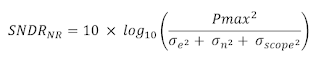Figure 6. SNDRNR equation with oscilloscope noise removed.

Teledyne LeCroy's PCIe 6.0 Base Transmitter compliance test solution implements several noise-removal methodologies. The bottom of Figure 6 shows two measurements that were made on a Teledyne LeCroy LabMaster oscilloscope. The first is the SNDR without oscilloscope noise removed, and the second is the SNDRNR measurement with the oscilloscope noise removed.

### RLM Measurement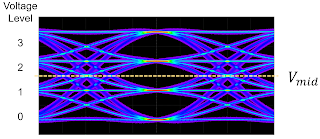Figure 7. V0, V1, V2 and V3 signal levels for calculating the transmitter RLM.
Transmitter linearity is defined as a function of the mean signal levels V0, V1, V2 and V3 transmitted for PAM4 two-bit symbols, as shown in Figure 7.

Transmitter RLM is defined by the following equations involving V0, V1, V2 and V3:

Vmid = (V0 + V3) / 2

ES1 = (V– Vmid) / (V0 – Vmid)

ES2 = (V2 – Vmid) / (V– Vmid)

RLM = min((3 x ES1 ), (3 x ES2 ), (2 – (3 x ES1 )), (2 – (3 x ES2 )))

RLM is measured using the same section of the Compliance Pattern signal used for 𝜎n for the SNDR measurement, which is the 64-UI-long runs of 0s, 1s, 2s and 3s. The RLM measurement is straightforward. The goal is to have evenly spaced transition levels and to have RLM equal to 1.

### ps21TX Measurement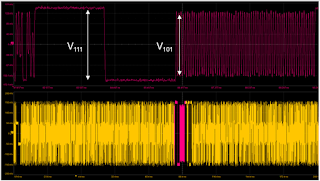Figure 8. ps21TX measurement.
A key voltage measurement to make for PCIe 6.0 Base transmitter testing is the ps21TX measurement—the effective transmitter package loss. This measurement was also defined in the PCIe 5.0 Base specifications, so it's not a new measurement for PCIe 6.0. The package loss is measured by comparing the 64 0s and 64 1s voltage swing against a 1, 0, 1, 0 clock pattern. The ps21TX measurement is conducted with no transmitter equalization, and it is made by averaging over 500 repetitions of the Compliance Pattern signal to reduce noise. The measurement is illustrated in Figure 8, where V111 is the amplitude of a low-frequency signal and V101 is the amplitude of a high-frequency signal. The ps21TX measurement is then defined as follows:

ps21TX  = 20 × log10 (V101 / V111 )

Adding the SDAIII-PAMx and SDAIII-PCIE6 options to Teledyne LeCroy oscilloscopes provides all the software tools needed to easily make the new Compliance Pattern measurements to the PCIe standard, including the required oscilloscope noise removal. We'll discuss the noise removal methods in our next post.